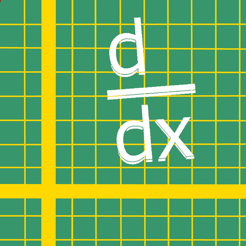## iPhone Screenshots

•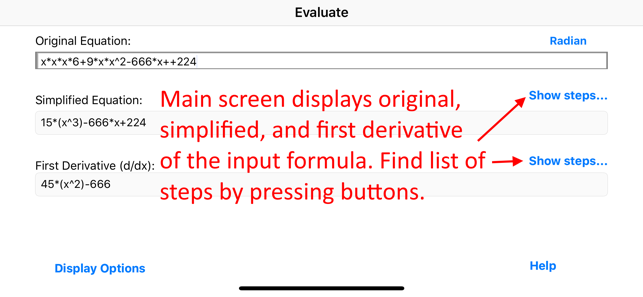••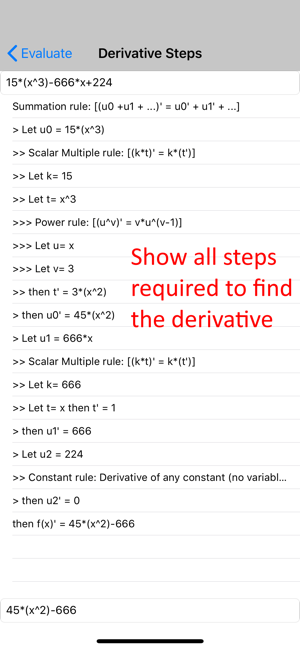•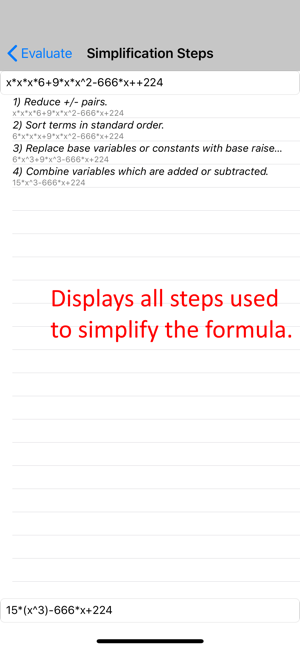•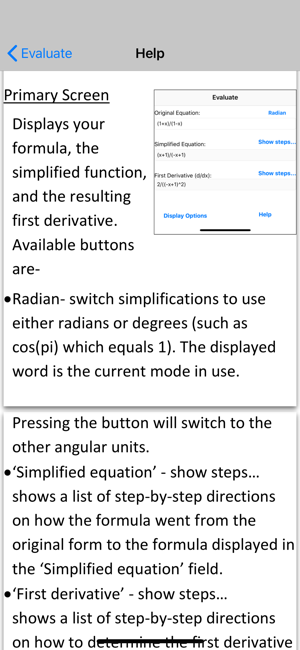## Description

uDerivative is a fast and simple way to learn how to simplify functions, and, to calculate and learn derivatives. You control the level of detail in calculating the derivative, the number of significant digits, and whether you want to use symbols (pi/e) or values.

Enter your formula with the calculator and the formula is simplified and displayed along with the resulting derivative. Press a button and get the steps to simplify the original function, press another button and learn how to calculate the derivative.

The steps are shown in a simple step-by-step list format. The simplified formula is calculated using basic algebra, each step describes what was done and the formula after that step is completed. The steps to determine the derivative are also available in a separate window. These use a tree-view format so you can easily collapse sub-steps out of the way making the rest of the solution easier to understand.

Features:

2) Supports all four orientations of your device.
3) Main screen-
a. Displays input formula.
b. Switch between radians and degrees.
c. Display simplified equation.
d. Display first derivative of your formula.
e. Display options to modify how calculations are performed.
f. Local help to assist you in using and understanding the calculus involved.
4) Simple input using a calculator style input-
a. Supports standard cut and paste operations.
b. Supports variable ëxí.
c. Standard operators for add, subtract, multiply, divide, raise to a power (+, -, *, /, ^).
d. Parenthesis to group operations.
e. Supports all trig functions and their inverse trigonometric functions.
f. Supports hyperbolic trigonometric functions and their inverse functions.
g. Symbolic pi and e, you can display them as numbers if you want.
h. Natural log and log base-10.
i. Square-root, sign, raising e or 10 to a power.
j. Sign function (if contents are positive or negative).
k. Absolute value.
5) Formula simplifications-
a. Displays final simplified answer on main screen.
b. Step-by-step derivation available by pressing a button.
c. Each step describes the algebraic formula and the function after the operation.
6) Calculation of first derivative-
a. Final answer displayed on screen.
b. Step-by-step calculation of derivative shown by pressing a button.
c. All rules covered and described, listing is hierarchical for proper sub-step illustration.
d. Tree-view display allows easy method to remove sub-steps from main calculations.
d. Level of detail controlled through display options.
7) Display options-
a. Control detail level in derivatives (verbose, medium, terse).
b. Control how many digits of precision to display in the final answers.
c. Whether or not to convert pi and e to values, or, to keep as symbols.
8) Help-
a. How to use the program.
b. Description of available functions and operations with examples of use.
c. Derivatives- description of basic rules and full tables of derivatives.

## What’s New

Version 2.01

Better formula simplification
Minor bugs

## Ratings and Reviews

Mathkid101 ,

### Terrible

I took a shot with this app since there were no reviews when I downloaded it. I should have listened to my gut and kept searching. You get what you pay for... A 99 cent app with little to no interaction. You're better off without it

## Information

Seller
Creative Mindset Software, LLC
Size
2.7 MB
Category
Utilities
Compatibility

Requires iOS 9.0 or later. Compatible with iPhone, iPad, and iPod touch.

Languages

English

Age Rating
4+
Price
\$0.99

## Supports

•